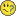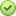# QlikView Scripting

Discussion Board for collaboration on QlikView Scripting.

Announcements

#### Breathe easy -- you now have more time to plan your next steps with Qlik! QlikView 11.2 Extended Support is now valid through December 31, 2020. Click here for more information.Not applicable

## Sum of Costs in current week in Textbox

Hello everyone

I would like to show the sum of my Field "costs" in a textbox. But only of the current week. What is the expression for this?

Thank you very much in advance...

Tags (1)
1 Solution

Accepted Solutions
Not applicable

## Re: Sum of Costs in current week in Textbox

true so add year in the set expression as well=Sum({<Week={"\$(=week(today()))"}, Year={"\$(=Year(today()))"}>} costs)

12 Replies
Not applicable

## Re: Sum of Costs in current week in Textbox

=Sum({<Week={"\$(ThisWeekVariable)"} >} costs)

Assuming that you have a variable holding this week

or you may replace the variable with an expression for this week :

=Sum({<Week={"\$(=week(today()))"} >} costs)Not applicable

## Re: Sum of Costs in current week in Textbox

Hi,

Try something like this

=sum( {\$<Week = Week(today()) >} Costs )Not applicable

## Re: Sum of Costs in current week in Textbox

Ευχαριστώ

But then I get all values from week 40, also in the years before, right?

Best

Jan

Not applicable

## Re: Sum of Costs in current week in Textbox

true so add year in the set expression as well=Sum({<Week={"\$(=week(today()))"}, Year={"\$(=Year(today()))"}>} costs)Not applicable

## Re: Sum of Costs in current week in Textbox

Okay, the value in the textbox is still the total sum of all values.The whole expression is underlined with a red line. something does not fit with the expression yet...

Not applicable

## Re: Sum of Costs in current week in Textbox

Try like this

=Sum({<Week={"\$(=week(today()))"},Year={"\$(=Year(today()))"} >} costs)

Replace fields with your actual fields

Regards

ASHFAQNot applicable

## Re: Sum of Costs in current week in Textbox

AWESOME! Thank you very much!Not applicable

## Re: Sum of Costs in current week in Textbox

Try this

Sum({\$<DATE={"<=\$(=Date(Max(DATEWeek)-7,'DD-MM-YYYY'))"}>}costs)

Make sure Date format is same as used for Date field.

Not applicable

## Re: Sum of Costs in current week in Textbox

Hi,

Can you close this thread by selecting appropriate answers.

Regards

ASHFAQ

Community Browser